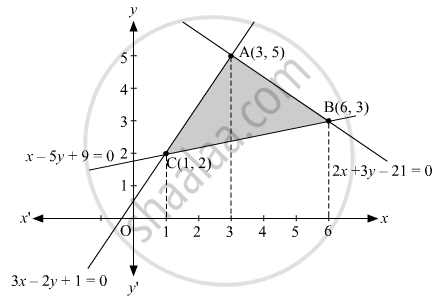# Using the method of integration, find the area of the region bounded by the lines 3x − 2y + 1 = 0, 2x + 3y − 21 = 0 and x − 5y + 9 = 0 - Mathematics

Sum

Using the method of integration, find the area of the region bounded by the lines 3x − 2y + 1 = 0, 2x + 3y − 21 = 0 and x − 5y + 9 = 0

#### Solution

3"x" - 2"y" + 1 = 0 ⇒ "y"_1 =((3"x"+1))/2 ...........(i)

2"x" - 3"y" - 21 = 0 ⇒ "y"_2 =((21-2"x"))/3 .....(ii)

"x" - 5"y" + 9 = 0 ⇒ "y"_3 = (("x"+9))/5 ......(iii)

Point of intersection of (i) and (ii) is A(3, 5)

Point of intersection of (ii) and (iii) is B(6, 3) and

Point of intersection of (iii) and (i) is C(1, 2).Therefore, the area of the region bounded=int_1^3"y"_1."dx"+int_3^6"y"_2."dx"-int_1^6"y"_3". dx"

=int_3^1((3"x"+1))/2."dx"+int_3^6((21-2"x"))/3."dx"-int_1^6(("x"+9))/5."dx"

=1/2((3"x"^2)/2+"x")_1^3+1/3(21"x"-"x"^2)_3^6-1/5("x"^2/2+9"x")_1^6

1/2+1/3-1/5[125/2]

= 7+12-12.5

= 6.5  "sq.units"

Concept: Area of the Region Bounded by a Curve and a Line
Is there an error in this question or solution?